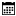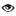s

#### AutoMapper

Murugan Andezuthu Dharmaratnam |10 September 2020 |4458

It's easy to remove duplicates from a single dimensional array. The sample code below removes duplicates from a list of objects. The code will be able to remove duplicate Name, It does not compare all properties of the object. The first Id will be taken and the remaining will be ignored.

## SOLUTION 1

```public List GetFilteredList()
{
List lstcountries = new Country().Get();
List lstFiltered = new List();
foreach(var item in lstcountries)
{
if(!lstFiltered.Where(x => x.Name == item.Name).Any())
{
}
}

return lstFiltered;
}
```

## Code For Country Class & Data

```public class Country
{
public int Id { get; set; }
public int Code { get; set; }
public String Name { get; set; }

public List Get()
{
List<Country> Countries = new List<Country>
{
new Country() { Id = 1, Name="India", Code = 91 },
new Country() { Id = 2, Name="China" , Code = 86},
new Country() { Id = 3, Name="USA" , Code = 1},
new Country() { Id = 4, Name="India" , Code = 91},
new Country() { Id = 5, Name="Russia" , Code = 7},
new Country() { Id = 6, Name="India" , Code = 91},
new Country() { Id = 7, Name="China" , Code = 86}
};
return Countries;
}
}
```

Output of code would be

```Id = 1, Name="India", Code = 91
Id = 2, Name="China", Code = 86
Id = 3, Name="USA", Code = 1
Id = 5, Name="Russia", Code = 7
```
The code takes the first Id as it appears on the list and it will not take the remaining Id.

## Solution 2

```lstcountries.Select(x => new {  x.Name, x.Code} ).Distinct().ToList()
```

This solution is if you do not want the Id, Any way Id will not be useful . Problem with the above code is it does not have an Id. Check the below code which will save the output to a list of Country. Please note all Id's in the below code will be 0

```List<country> lstFiltered = lstcountries.Select(x => new { x.Name, x.Code }).Distinct().Select(y => new Country() { Code = y.Code, Name = y.Name, Id = 0 }).ToList();
```